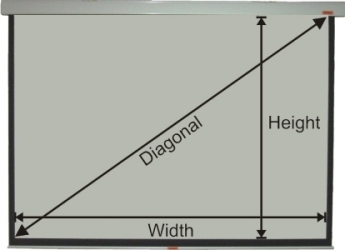﻿ Images Size Calculator
Image Size Calculator
Image Size Calculator
 Use the calculator below to determine image dimensions: Video Format ( Ratio 4:3 or 1:1.33 ) HDTV Format ( Ratio 16:9 or 1:1.778 ) Wide Screen ( Ratio 1:1.85 ) Cinema Scope ( Ratio 1:2.35 ) Select Screen Format: Video Format HDTV Format Wide Screen Cinema Scope Select Unit: mm cm inches feet Enter Width, Height or Diagonal length to calculate measurements for other two dimensions. Height Diagonal Unit Width Width Diagonal Unit Height Width Height Unit Diagonal